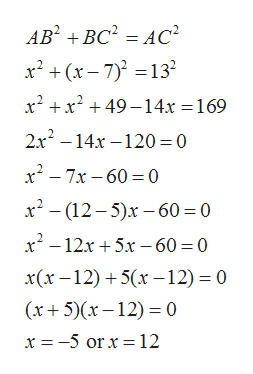show steps pleasethe length of one leg of a right triangle is 7 meters less than the other leg. the length of the hypotenuse is 13 meters. find the lengths of the legs.

Question

the length of one leg of a right triangle is 7 meters less than the other leg. the length of the hypotenuse is 13 meters. find the lengths of the legs.

Step 1

Given that the length of one side in right angle triangle is 7 meters less than the other leg. Suppose the length of side AB is x meter then length of side BC is (x- 7) meter and length of hypotenuse is 13 meter as shown in the below figure

Step 2

Since ABC is right angle triangle...help_outlineImage TranscriptioncloseAB BC AC2 x2x-7)13 x2x249-14x = 169 2x? -14х — 120 %3D0 x27x 60 0 x2- (12-5)x-60 0 x12x5x -60 = 0 x(х -12) + 5(х —12) — 0 (x5)x12)0 x =-5 orx= 12 fullscreen

Want to see the full answer?

See Solution

Want to see this answer and more?

Our solutions are written by experts, many with advanced degrees, and available 24/7

See Solution
Tagged in

Other